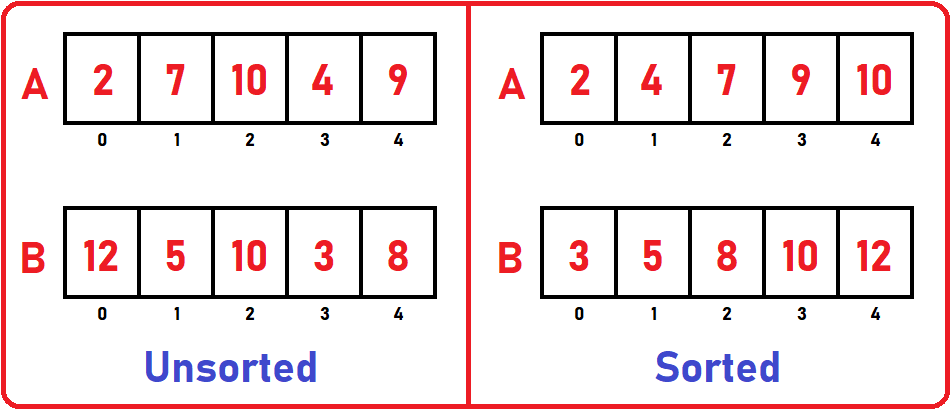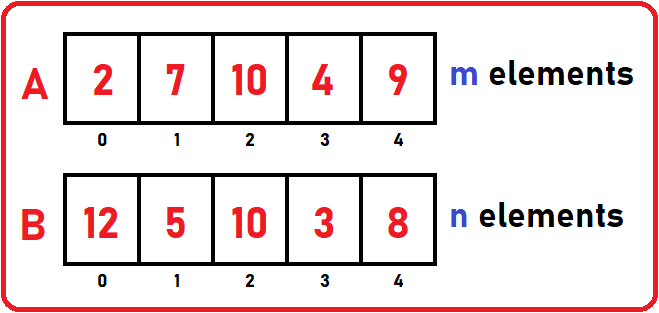# Array Set Operations in C

## Array Set Operations in C Language with Examples:

In this article, we will see Array Set Operations in C Language with Examples. In our previous article, we have discussed Merging two Arrays with Examples.

##### Array Set Operations in C

First, let’s see what are set operations. We will cover the following set operations in our article:

1. Union – It will combine the two arrays such that the final set will not contain any duplicate elements. It is similar to merging.
2. Intersection – It will point to common elements between two arrays and copy those elements in a 3rd array.
3. Difference
4. Set Membership

Below is the example of two sets that are stored in arrays A and B:If the set is stored in an array, then how we can perform these operations. Let’s see different operations in sorted and unsorted arrays.

##### Union Set Operation in Array:

Let’s say array A has m elements and array B has n elements.Now the procedure for union is:

We have to create a 3rd array of the name ‘C’. Its size will be the size of array A + size of array B. Then copy all the elements of the first array to our 3rd array C:The first array is copied to array C. How much time is taken? m time. Now, should we copy all elements of array B? We cannot blindly copy 2nd set. If any element of 2nd set is already present in array C then we have to leave that element and check for the next one. i.e. Is 12 presents in set C? No, copy 12 to C:Check next element, Is 5 present in set C? No, copy 5 to C:Check next, Is 10 presents in set C? Yes, so don’t copy 10. In the same manner rest of the elements of set B will be copied in Set C:Depending on the number of elements, the search will take m time, and for how many times we have done this? For n times. So total time is:We will not take 2 variables; we will take only a single variable. So, let’s modify the above statement as:

Time Complexity: n + n * n = n + n= O (n2)

So, for performing union it has taken n2 time. This is quadratic and very slow. We have seen union in the unsorted set. Now let’s see union operation in sorted sets:Now we want to apply union operation in the above 2 sorted sets or lists. Set A has m elements and set B has n elements. Let’s create a set C where we want to store combined elements without duplicates of sets A and B. Its size will be the size of set A + the size of set B. so we can use the merge procedure here. We have already discussed merging in our previous article, that same merging procedure we have to perform here.

For merging, we need to use index pointers. We are not explaining the full process of merging here but just giving the overview of merging. So, we will initialize i to A , j to B , and k to C :We will compare each element of set A to every element of set B and which one will be smaller, copy that to C of k, and increment that set index pointer and k this is the process of merging. Those elements which will be present on both sets, we will copy them only once. After merging, we will get:So, in this way we have performed union operations in sorted sets.

Time Complexity: θ (m + n)

If we want to represent this in a single variable then: Time Complexity: θ (n+ n) = θ (n)

Now let’s look at the Intersection operation of the set.

##### Intersection Set Operation in Array:

In this operation, we have to take only common elements from set A and B and copy those common elements into a separate set. We have:We will perform intersection on both sorted and unsorted sets and will see which ones take less time. Let’s first see in unsorted set:

So, the procedure is to start checking the elements from set A. Check if A  is present in set B, if no, point to the next element and if yes copy it to set C. In our case 2 is not present in set B so point next to one. Now check if A  is present in set B, no, again leave it and point to the next element. Check for if A  is present in set B, yes, copy it to set C.Now there is no common elements between set A and set B. As we have to compare every element of set A to every element of set B, it will take O (n*n) time to perform intersection on two sets. So,

Time Complexity: O (n)

Now let’s see intersection operation in sorted sets A and B:Here also we use the same procedure of merging. Again, we will use index pointers. So, we will initialize i to A , j to B , and k to C :Here we have to do some changes in our algorithm of merging. At the time of comparing elements of set A and B, we will increment that index pointer which element is smaller compared to other set elements.

For example, in our case, A [i] is smaller than B [j] so don’t copy any of the elements just increment index pointer i because its element is smaller. Then again check for which element is smaller A [i] or B [j], then increment that set’s index pointer by one. We have to copy only those elements which will be common in both sets.

In the above two sets, only one element is common – 10, so copy only 10 to set C:Time Complexity: θ (m + n)

In single variable: Time Complexity: θ (n+ n) = θ (n)

##### Difference Set Operation in Array:

We have two sets then we want to perform difference operation on them such as A – B that is the subtraction of two sets. It means we want all those elements of set A which are not present in set B. So, subtract the common elements between sets A and B. Take only those elements which are only in set A but not in set B. This is difference operation. Let’s perform this operation in below two sets:We can clearly see in the above sets, 2, 7, 4, and 9 are elements which only present in set A not in B. So, what is the procedure for this? We have to check every element of A in B and check if two elements are the same then move to next, don’t copy any element but if two elements are different then copy the elements of set A to set C:Time Complexity: O(n2)

Above is the example of an unsorted list, let’s see difference operations in the sorted list:In sorted array, we again use the process of merging with some modifications. We will copy only those elements which are only in A, so again we will take index pointers as:We are not copying from B, we are copying from A, so we will copy smaller elements in A as compared to B and will stop as A list will be completely traced:Time Complexity: θ (m + n)

In single variable: Time Complexity: θ (n+ n) = θ (n)

##### Set Membership Set Operation in Array:

This operation is used to know whether the given element is belongs to that set or not.Is 5 belong to set A? Yes. Is 4 belong to set A? No. it is a normal search operation in an array. So, we have discussed all the 4 operations in this article, Now let’s see the code part for each and every operation:

##### Array Set Operations Full Code in C language:
```#include<stdio.h>
#include<stdlib.h>

struct List {
int B;
int size;
int length;
};

void Display(struct List list) {
int i;
printf("\nElements are\n");
for (i = 0;i<list.length;i++)
printf("%d ", list.B[i]);
}

struct List* Union(struct List *list1, struct List *list2) {

int i, j, k;
i = j = k = 0;

struct List *list3 = (struct List *)malloc(sizeof(struct List));

while (i < list1->length && j < list2->length) {
if (list1->B[i]<list2->B[j])
list3->B[k++] = list1->B[i++];
else if (list2->B[j]<list1->B[i])
list3->B[k++] = list2->B[j++];
else {
list3->B[k++] = list1->B[i++];
j++;
}
}

for (;i<list1->length;i++)
list3->B[k++] = list1->B[i];
for (;j<list2->length;j++)
list3->B[k++] = list2->B[j];

list3->length = k;
list3->size = 10;

return list3;
}

struct List* Intersection(struct List *list1, struct List *list2) {
int i, j, k;
i = j = k = 0;

struct List *list3 = (struct List *)malloc(sizeof(struct List));

while (i < list1->length && j < list2->length) {
if (list1->B[i] < list2->B[j])
i++;
else if (list2->B[j] < list1->B[i])
j++;
else if (list1->B[i] == list2->B[j]) {
list3->B[k++] = list1->B[i++];
j++;
}
}

list3->length = k;
list3->size = 10;

return list3;
}

struct List* Difference(struct List *list1, struct List *list2) {
int i, j, k;
i = j = k = 0;

struct List *list3 = (struct List *)malloc(sizeof(struct List));

while (i<list1->length && j<list2->length) {
if (list1->B[i]<list2->B[j])
list3->B[k++] = list1->B[i++];
else if (list2->B[j]<list1->B[i])
j++;
else {
i++;
j++;
}
}

for (;i<list1->length;i++)
list3->B[k++] = list1->B[i];

list3->length = k;
list3->size = 10;

return list3;
}

int main() {
struct List list1 = { { 2, 4, 7, 9,10 },10,5 };
struct List list2 = { { 3, 5, 8,10,12 },10,5 };
struct List *list3;

printf("First Set:");
Display(list1);

printf("\n\n");

printf("Second Set:");
Display(list2);

printf("\n\nDifference Operation on above 2 sets:");
list3 = Difference(&list1, &list2);
Display(*list3);

return 0;
}
```
###### Union Operation Output:###### Intersection Operation Output:###### Difference Operation Output:In the next article, I am going to discuss Menu Driven Program using Array in C Language with Examples. Here, in this article, I try to explain Array Set Operations in C Language with Examples and I hope you enjoy this Array Set Operations in C Language with Examples article.